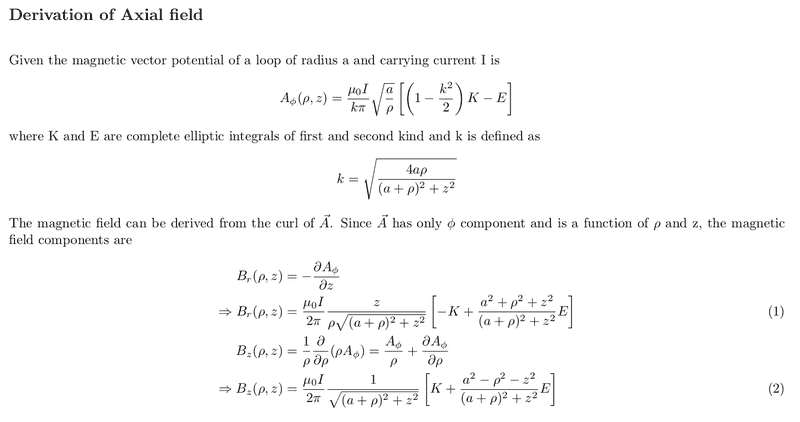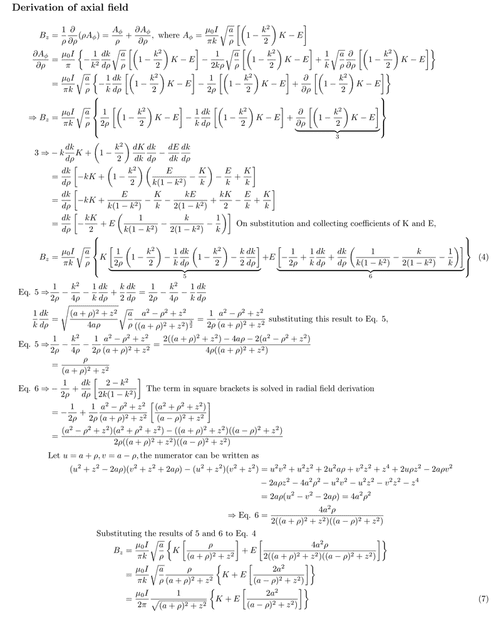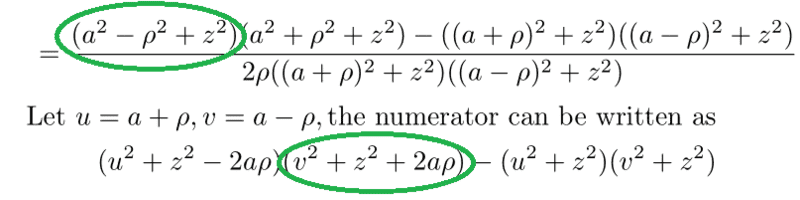# Calculate the magnetic field from the vector potential

• arjun_ar
In summary, the conversation discusses the derivation of radial and axial magnetic fields of a current carrying loop from its magnetic vector potential. The speaker has successfully derived the radial field but is facing difficulties with the axial field derivation. They share their derivation of the radial field and ask for help in identifying where they went wrong in the derivation of the axial field. They also inquire about the independence of certain variables in the equations and point out a potential mistake. The responder confirms the mistake and suggests a correction for equations (1) and (2). f

#### arjun_ar

Homework Statement
Given the magnetic vector potential of a current carrying loop in cylindrical coordinate system, derive the axial and radial magnetic fields.
Relevant Equations
I am trying to derive radial and axial magnetic fields of a current carrying loop from its magnetic vector potential. So far, I have succeeded in deriving the radial field but axial field derivation gives me trouble.My derivation of radial field (eq 1) can be found here.

Can anyone point out where I went wrong in the derivation of axial field?

My derivation of axial field is given below.In case the images are blurred, you can see them here and here.

On comparing, Eq.7 with Eq.2, the coefficient of E do not match.

I have done this derivation multiple times, yet arrive at the same answer.
Can anyone point me where I went wrong?

Last edited:
•Delta2
Hmm is it dead sure that K and E are independent of ##\rho,z##? So you can treat them as constants when taking the derivatives with respect to those variables?

•arjun_ar
I think the mistake is here:The two expressions circled in green are not equivalent.

-----------------------------------------------------------------------

Do you have typographical errors in your equations (1) and (2)? Should the denominators inside the square brackets be ##(a-\rho)^2 + z^2## instead of ##(a+\rho)^2 + z^2##?

••arjun_ar and Delta2
Hmm is it dead sure that K and E are independent of ##\rho,z##? So you can treat them as constants when taking the derivatives with respect to those variables?
I think they are independent of ##\rho,z##. In my derivation of radial field, I have treated them to be independent of ##z## and arrived at the exact solution.

•Delta2
I think the mistake is here:

View attachment 304311

The two expressions circled in green are not equivalent.

-----------------------------------------------------------------------

Do you have typographical errors in your equations (1) and (2)? Should the denominators inside the square brackets be ##(a-\rho)^2 + z^2## instead of ##(a+\rho)^2 + z^2##?
Thank you! This solves my problem. I don't know how I missed it!

There is indeed a typographical error for equations (1) and (2). I will update them and provide a full derivation for axial field asap.

•TSny and Delta2